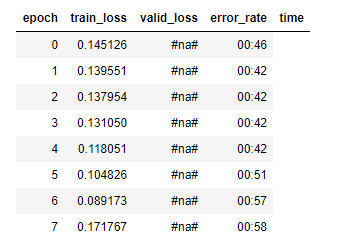# 📝 Deep Learning Lesson 2 Notes

Detailed lesson 2 notes by Hiromi.

2 Likes

While picking a learning rate, when do we pick 3e-5 vs 1e-5 ? There was a part of the video in lesson 2 where Jeremy saw the image and put 3e-5 and I wasn’t sure when 1e-5 would be used against 3e-5

1 Like

After download the url on the local , I have to uplaod that url on the kaggle . The directory created by the python code is “data/beers/…” , then how to upload the file from local to this directory location , because from the “Add Dataset” option on the kaggel , dosent show me this location. Please help me .

`urls = Array.from(document.querySelectorAll('img.tile--img__img.js-lazyload')).map(el=>decodeURIComponent(el.src.split('='))); window.open('data:text/csv;charset=utf-8,' + escape(urls.join('\n')));`

3 Likes

I created SGD for square functions. I hope this is the right place to post it
https://www.kaggle.com/matejthetree/sgd-2?scriptVersionId=15140809
I am bad with plot, so I dont know why it pops two images at the endI manged to download some images of different Chinese Calligraphy artworks. Plan is to build a Chinese calligraphy style classifier. I did all the work on a Kaggle Kernel and uploaded images as private dataset to be used by the kernel. My question may be quite dumb, but what kind of license should I use for the dataset? Or should I upload to Kaggle at all without breaking some license? I want to share the notebook on Kaggle once I finished, but if license won’t clear, I don’t think I can do that. Anyone here could shed some lights on this topic? Much appreciated!

Does anyone know why my epochs always start from zero instead of one (when I run learn.fit_one_cycle)?
Also why Jeremy used max_lr slice (3e-5, 3e-4) instead of (1e-5, 1e-4) (after running learn.recorder.plot())?

Can Some one please turn this into a set of equations, Especially the

``````loss.backward()
``````

I’m having a hard time understanding it.

1 Like

a : is the weight tensor (that also stores its gradient in a.grad ) that our model will determine during the training of our model (y = x @ a)
a.grad is an attribute of the a tensor where the gradient of a is stored

a.grad is calculated after each call of the loss.backward() function
Then a is update like this
a = a - lr * a.grad
which can be written like this
And in pytorch, it is written like this
it’s called in-place sub() because it directly updates the a tensor in-place

(by the way, if you see a function that ends with `_` like sub_(), it means it’s an in-place version of its correspondent function (like add and add_) : It’s a convention)

Once we finish updating the a tensor, we have to reset a.grad to zero ( a.grad.zero_() ) before calling the next loss.backward() function.

As for the with torch.no_grad(): , we use it to ask pytorch to stop updating (tracking) a.grad (it’s already calculated after the loos.backward() call) while we are updating the a tensor

2 Likes

Thanks a lot, i spent like 30 mins trying to figure out why i couldnt download. Appreciate it!

Hi all,

I have one quick question regarding the `update()` function. Been trying to wrap my head around this concept.

Question:

How do we know that if we move the whole thing downwards, the loss goes up and vice versa?

Appreciate any insights. Thank you.

I believe the learned coefficients in the linear recession example will tend to (3,2.5).

So, I am using Mozilla Firefox, and it seems I can download the links using the code in the tutorial, but am I supposed to save the file in a particular directory? Or is there a different javascript for Firefox to download the links?

Hello. I know this reply is a bit late but it may still be of help to someone else. If i understand, you’re confused about why the loss goes up when the whole thing is moved downwards. Think of it this way, under normal conditions, when the gradient of a quadratic function is taken, it gives us the direction that increases the loss. Instead of increasing the loss, we desire the loss to be decreased so what we do is to take the negative of the gradient.

I saw a pretty good explanation here. https://medium.com/@aerinykim/why-do-we-subtract-the-slope-a-in-gradient-descent-73c7368644fa

I was confused because I didn’t realise that (1) he was referring to the gradient of the loss func and (2) I couldn’t visualize it until someone drew it out clearly

Hi all,

I’m having trouble after cleaning my images and training the model with the new databunch created from the new cleaned csv.
When getting the learn rate (learn.lr_find()), im getting #na# values instead of valid_lossess. It seems columns are displazed to the left as instead or error_rate im getting in that column the time.This is normal, `valid_loss` and `error_rate` are not calculated by the learning rate finder. It only checks for the loss on the test set, this is because our aim is to determine the learning rate using lr_find() and not to train the model. (that will be done later using `learn.fit_one_cycle()`)

Oh i thought that lr_find calculates the valid_loss and the error_rate for each lr it tries out.
Then why is the time column in the error_rate column?
Thanks!

I think you see the time values below error_rate because the error_rate value is just null and 0 characters long so the next value is displayed just after.

Hello.
Is Stochastic gradient descent technique same as Stochastic deep learning??